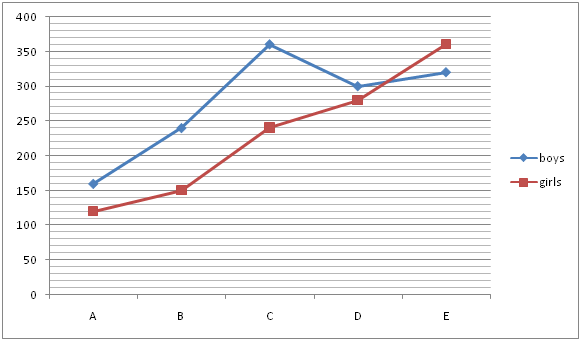# IBPS Clerk Prelims Quantitative Aptitude Questions 2019 (Day-12)

Dear Aspirants, Our IBPS Guide team is providing new series of Quantitative Aptitude Questions for IBPS Clerk Prelims 2019 so the aspirants can practice it on a daily basis. These questions are framed by our skilled experts after understanding your needs thoroughly. Aspirants can practice these new series questions daily to familiarize with the exact exam pattern and make your preparation effective.

Check here for IBPS Clerk Prelims Mock Test 2019

[WpProQuiz 7346]

Ensure Your Ability Before of Exam – Take IBPS Clerk Prelims Free Mock test

Missing number series

Directions (01 – 05): What value should come in the place of (?) in the following number series?

1) 12, 40, 106, ?, 340, 352

a) 240

b) 200

c) 210

d) 220

e) 250

2) 1, 5, 32, 48, 173, ?

a) 222

b) 516

c) 209

d) 389

e) 427

3) 340, 510, ?, 1147.5, 1721.25, 2581.875

a) 765

b) 775

c) 785

d) 795

e) 755

4) 19, 30, 63, 118, 195, ?

a) 286

b) 290

c) 294

d) 298

e) 308

5) 20, 74, 112, 136, 148, ?

a) 150

b) 156

c) 162

d) 168

e) 164

Data Interpretation

Directions (6 – 10): Study the following information carefully and answer the questions given below.

The given line graph shows the number of boys and girls in five different schools in 2018.6) What is the ratio of the number of boys from D to number of girls from C?

a) 3: 4

b) 4: 3

c) 5: 4

d) 4: 5

e) None of these

7) Number of girls from B is approximately what percent of the total number of students from E?

a) 20%

b) 22%

c) 24%

d) 26%

e) 28%

8) What is the difference between the total number of students from C and A?

a) 280

b) 300

c) 320

d) 340

e) 360

9) What is the ratio of the average number of boys to girls from all the schools together?

a) 4:5

b) 5:4

c) 6:5

d) 7:6

e) None of these

10) What is the difference between the total number of students from D and B?

a) 120

b) 150

c) 170

d) 190

e) 210

Directions (1-5) :

12 * 3 + 4 = 40

40 * 2.5 + 6 = 106

106 * 2 + 8 = 220

220 * 1.5 + 10 = 340

340 * 1 + 12 = 352

1 + 22 = 5

5 + 33 = 32

32 + 42 = 48

48 + 53 = 173

173 + 62 = 209

340 * 1.5 = 510

510 * 1.5 = 765

765 * 1.5 = 1147.5

1147.5 * 1.5 = 1721.25

1721.25 * 1.5 = 2581.875

19 + (1* 11) = 30

30 + (3 * 11) = 63

63 + (5 * 11) = 118

118 + (7 * 11) = 195

195 + (9 * 11) = 294

20 + (82 – 10) = 74

74 + (72 – 11) = 112

112 + (62 – 12) = 136

136 + (52 – 13) = 148

148 + (42 – 14) = 150

Directions (6-10) :

Required ratio = 300: 240

= 5: 4

Required percentage = [150/(320 + 360)] * 100

= 22%

Total number of students from A = 160 + 120 = 280

Total number of students from C = 360 + 240 = 600

Difference = 600 – 280 = 320

Average boys = (160 + 240 + 360 + 300 + 320)/5

= 276

Average girls = (120 + 150 + 240 + 280 + 360)/5

= 230

Required ratio = 276:230

= 138:115

= 6:5